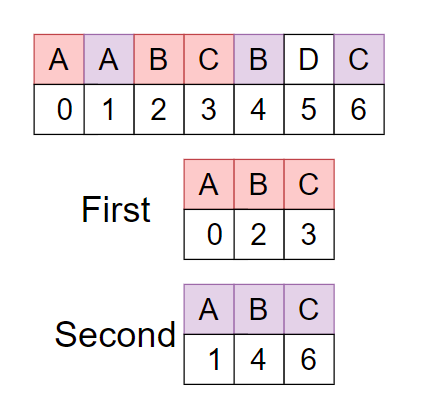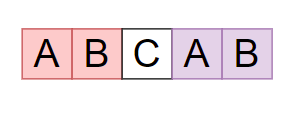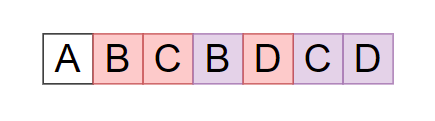7

# Longest Repeating Subsequence

Difficulty: MEDIUMContributed By
Sakshi Bansal
Avg. time to solve
15 min
Success Rate
85%

Problem Statement
Suggest Edit

#### You are given a string ‘st’, Your task is to find the length of the longest repeating subsequence such that two subsequences don’t have the same character at the same position.

##### For Example :
``````The given string st = AABCBDC.
````````````As you can see there are two repeating longest subsequences “ABC” with the same character but different position. Therefore the required answer is ‘3’ as the length of “ABC” is 3.
``````
##### Input Format :
``````The first line of input contains an integer ‘T’ denoting the number of test cases.

The first line of each test case contains a single integer ‘N’, where ‘N’ denotes the length of string ‘st’.

The second line of each test case contains a string ‘st’.
``````
##### Output Format :
``````For every test case, print the length of the longest repeating subsequence.

Output for each test case will be printed in a separate line.
``````
##### Note :
``````You do not need to print anything; it has already been taken care of. Just implement the given function.

All the characters of the given string ‘st’ are uppercase letters.
``````
##### Constraints :
``````1 <= T <= 50
1 <= N <= 100

Time Limit: 1 sec
``````
##### Sample Input 1 :
``````2
5
ABCAB
7
ABCBDCD
``````
##### Sample Output 1 :
``````2
3
``````
##### Explanation of Sample Input 1 :
``````Test Case 1

Given string ‘st = ABCAB’
````````````As you can see longest repeating subsequence is ‘AB’
So the length of ‘AB’ =2.

Test Case 2

Given string ‘st = ABCBDCD’
````````````As you can see longest repeating subsequence is ‘BCD'
Return length of ‘BCD’ = 3.
``````
##### Sample Input 2 :
``````2
2
BA
4
BCCB
``````
##### Sample Output 2 :
``````0
1
``````Console